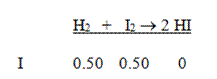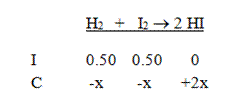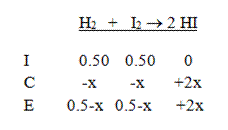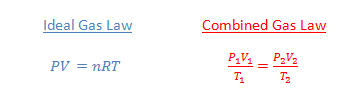## SI unit prefixes

Even with scientific notation, very large numbers and very small numbers can be awkward to work with. That is why scientists have developed a set of standard prefixes that are attached to a unit to indicate multiples of that unit. Each of the prefixes represents a particular power of ten. There are currently 20 prefixes in use:

Prefixes greater than 1

 Factor Name symbol 1024 yotta Y 1021 zetta Z 1018 exa E 1015 peta P 1012 tera T 109 giga G 106 mega M 103 kilo k 102 hecto h 101 deka da

Prefixes less than 1

 Factor Name symbol 10-1 deci d 10-2 centi c 10-3 milli m 10-6 micro μ 10-9 nano n 10-12 pico p 10-15 femto f 10-18 atto a 10-21 zepto z 10-24 yocto y

Do you have to memorize all of these? No. If you are a high school chemistry or physics student, you should know the prefixes in blue above. If you are an AP chemistry or AP physics student, you should also learn the prefixes in red. If you plan to be a scientist or an engineer, it wouldn’t hurt you to learn the rest, but the extreme prefixes are not so common.

Why do the prefixes skip three powers of 10? This allows us to use numbers that are always between 1 and 999. This is easiest to explain with an example. Let’s say we are measuring very small electrical currents. The following table shows how we would state the values without scientific notation, with scientific notation, and with prefixes. You can see how convenient this prefix method is:

 Current Scientific notation Prefix notation 0.50 A 5.0 x 10-1 A 500 mA 0.050 A 5.0 x 10-2 A 50 mA 0.0050 A 5.0 x 10-3 A 5 mA 0.00050 A 5.0 x 10-4 A 500 μA 0.000050 A 5.0 x 10-5 A 50 μA 0.0000050 A 5.0 x 10-6 A 5 μA 0.00000050 A 5.0 x 10-7 A 500 nA 0.000000050 A 5.0 x 10-8 A 50 nA 0.0000000050 A 5.0 x 10-9 A 5 nA 0.00000000050 A 5.0 x 10-10 A 500 pA

## How to use ICE charts

When you are given the initial concentrations of the components in a reaction and you want to know the final concentrations at equilibrium, an ICE chart can be very useful. The ICE chart (some teachers call it an OCE chart or an IΔE chart) is a tool that helps you set up the proper algebraic expression in order to calculate the concentration change of each compound. It’s based on a very simple concept: the initial concentration of a compound plus the change in concentration of that compound must equal its final (equilibrium) concentration. To set up an ICE chart, follow these easy rules:

1. Write out the balanced equilibrium equation.
2. On the first line (I), put down the initial concentrations of each compound. If a compound is not in the initial mix, write 0. Be sure that you calculate the molarity, and not just the number of moles.
3. Pick a direction for the reaction to proceed. It’s usually pretty obvious, but if you aren’t sure which way the reaction will go, just choose one direction. It doesn’t matter if you guess wrong.
4. On the second line (C), use a variable to write the change in concentration for each compound using the balanced equation as a guide. For each compound that is consumed, write –Ax, where A is the coefficient of the compound in the balanced reaction. For each compound that is being formed, write +Ax. See the example below.
5. On the third line (E), write the sum of the first two lines. This must be the final concentration of each compound.
6. Use the equilibrium law and the value of Kc to write an expression with the equilibrium concentrations. Then solve this equation for x.
7. Use this value to determine the equilibrium concentrations of each compound. [Note: if the value of x is negative, this means you guessed wrong in step 3, and the reaction actually proceeds in the opposite direction. You will still get the correct final values of each compound if you add or subtract this negative value in this step.]

Example 1: 1.0 mole of H2 and 1.0 mole of I2 are placed in a 2.0 L flask. The following reaction takes place at 400°C: H2 (g) + I2 (g) → 2 HI (g). What are the concentrations of the three compounds at equilibrium? The Kc for the formation of hydrogen iodide (HI) from hydrogen and iodine gases is 64.1 at 400°C.

Solution: Start by writing the equation and the initial concentrations. Don’t forget to divide by the volume to find the molarities of all the compounds.Next, put in the changes in concentration in terms of x. This reaction must go to the right, and we get two moles of HI for each mole of the reactants:Then add the two columns to calculate to equilibrium concentrations:Finally, write the equilibrium expression and solve:$K_C=64.1= \dfrac{(2x)^2}{(0.5-x)^2}$$\sqrt{64.1} = \sqrt{\dfrac{(2x)^2}{(0.5-x)^2}}$$8.0= \dfrac{2x}{0.5-x}$$4-8x=2x$$4=10x$$x=0.4$$\therefore [ \text{H}_2]=[ \text{I}_2]=0.1 \, M; \, [ \text{HI}]=0.80 \, M$

Note that if you are given the change in concentration or the initial and final concentrations of a compound, you do not need to use an ICE chart. You can calculate the equilibrium values with a little bit of arithmetic. See the following example:

Example 2: The reaction in Example 1 is repeated at a different temperature with the same initial concentrations, and the final concentration of HI is determined to be 0.60 M. Determine the value of Kc at this new temperature.

Solution: To solve for Kc, we need to know the final concentrations of all three components. We are given the final value of HI, and knowing that we produced 0.60 M HI means that we must have lost 0.30 M of each of the reactants (use the balanced reaction to determine this). Since we started with 0.50 M of each, the final concentrations of each reactant must be 0.20 M (just subtract the numbers). So the equilibrium expression gives us:$K_C= \dfrac{(0.6)^2}{(0.2)(0.2)}=9.0$

## Balancing redox reactions in basic solutions

When balancing redox reactions, you must start by finding the number of electrons transferred in each half reaction. You usually can’t get the charges to balance if you don’t equate the electrons. In this post, I show you how to balance a redox reaction in a basic solution.

Example : Iodide ions are oxidized in a basic solution by permanganate ions to produce iodine and Mn2+ ions. Show the balanced reaction.

Solution: I’ve made this example a little harder than in my other redox reaction posts because we first need to figure out from the example what the half reactions look like. This is not as complicated as it sounds. Just follow the English and turn it into chemical formulas. Our problem should therefore look like this:

I + MnO4 → I2 + Mn2+

Now comes the tricky part. It turns out it isn’t very easy to balance a redox reaction in basic solutions by adding OH ions, because when we balance the hydrogen atoms, we throw off the balance of the oxygen atoms. So we employ a clever technique. We first balance the reaction as though it were an acidic solution, then we change the solution from acidic to basic. This is a five-step process, and you’ll notice the first four steps are identical to the process for balancing a redox reaction in an acidic solution:

1. Determine the number of electrons transferred in each half reaction, and balance the electrons.
2. Balance the oxygen atoms by adding water molecules.
3. Balance the hydrogen atoms by adding H+ ions.
4. Check you answer by verifying that the charges are balanced.
5. Cancel out the H+ ions by adding an equal number of OH ions to both sides, which changes the H+ ions into water.

Let’s see how this works on our example. The iodine atom goes from an oxidation number of 1- on the left to 0 on the right, so each iodine atom loses one electron. Since there are two iodine atoms in I2, we think of this half-reaction as losing two electrons. The manganese goes from an oxidation number of 7+ in the permanganate ion to an oxidation number of 2+. It has gained five electrons. To balance the electrons, we need a total transfer of ten electrons. Multiply the iodine compounds by five and the manganese compounds by two. (Note that the iodide ion was multiplied by 10 rather than 5 to balance the number of iodine atoms):

10 I + 2 MnO4 → 5 I2 + 2 Mn2+

Now we balance the oxygen atoms. There are 8 oxygen atoms on the left and none on the right, so we add 8 water molecules to the right side:

10 I + 2 MnO4 → 5 I2 + 2 Mn2+ + 8 H2O

Next we balance the hydrogen atoms by adding 16 H+ ions to the left (we pretend we have an acidic solution):

10 I + 2 MnO4 + 16 H+ → 5 I2 + 2 Mn2+ + 8 H2O

The equation should be fully balanced, and we check our work by verifying the charges balance. 10- (the iodide ions), 2- (the permanganate ions) and 16+ (the hydrogen ions) gives a net charge on the left of 4+. On the right side, we also have a total charge of 4+.

Finally, we are ready to change the reaction from acidic to basic. We cancel out the 16 H+ ions by adding 16 OH ions to both sides:

10 I + 2 MnO4 + 16 H+ + 16 OH → 5 I2 + 2 Mn2+ + 8 H2O + 16 OH

Because H+ and OH ions react to form water molecules, we simplify the left side:

10 I + 2 MnO4 + 16 H2→ 5 I2 + 2 Mn2+ + 8 H2O + 16 OH

Our last step is to simplify the equation by subtracting the 8 extra water molecules from the right side. We have to subtract the same number from the left side to keep the reaction balanced:

10 I + 2 MnO4 + 8 H2O → 5 I2 + 2 Mn2+ + 16 OH

(Look for other tips on this website if you need help with balancing simple redox reactions or redox reactions in acidic solutions.)

## Balancing redox reactions in acidic solutions

When balancing redox reactions, you must start by finding the number of electrons transferred in each half reaction. You usually can’t get the charges to balance if you don’t equate the electrons. In this post, I show you how to balance a redox reaction in an acidic solution.

Example 1: Balance the following reaction:

MnO4 + NO + H+ → Mn2+ + H2O + NO3

Solution: To balance reactions like this, use the following four-step procedure:

1. Determine the number of electrons transferred in each half reaction, and balance the electrons.
2. Balance the oxygen atoms by adding water molecules.
3. Balance the hydrogen atoms by adding H+ ions.
4. Check you answer by verifying that the charges are balanced.

In this reaction, the Mn atom goes from an oxidation number of 7+ in the permanganate ion to an oxidation number of 2+. It has gained five electrons. The nitrogen atom goes from an oxidation number of 2+ in the nitric oxide to 5+ in the nitrate ion. It has lost three electrons. To balance the electrons, we need to make the total number of electrons transferred equal 15 (because that’s the least common multiple of three and five!). We multiply the Mn ions by 3 and the N compounds by 5:

3 MnO4 + 5 NO + H+ → 3 Mn2+ + H2O + 5 NO3

The next step is to balance the oxygen atoms. There are 17 oxygen atoms on the left side of the reaction and 15 on the right side (before you include the water). Therefore we need two water molecules on the right:

3 MnO4 + 5 NO + H+ → 3 Mn2+ + 2 H2O + 5 NO3

Finally, we balance the hydrogen atoms (there are four of them in the water molecule on the right) by adding 4 hydrogen ions on the left:

3 MnO4 + 5 NO + 4 H+ → 3 Mn2+ + 2 H2O + 5 NO3

We check our work by verifying the charges balance. 3- (the permanganate ions) and 4+ (the hydrogen ions) gives a net charge on the left of 1+. 6+ (the manganese ions) and 5- (the nitrate ions) on the right also gives a net charge of 1+. We have succeeded!

Example 2: Complete and balance the following reaction. Assume it takes place in an acidic solution:

Cr2O72 + HSO3 → SO42 + Cr3+

Solution: In this reaction, the Cr atoms go from an oxidation number of 6+ in the dichromate ion to an oxidation number of 3+ in the chromate ion. Therefore, each chromium atom gains three electrons. But since there are two chromium atoms in the dichromate ion, we must think of this half-reaction as gaining six electrons overall. The sulfur atom goes from an oxidation number of 4+ to 6+, so it loses two electrons.

In order to balance the electrons, we need a net transfer of six electrons in each half-reaction. We multiply the ions with the sulfur atoms by three. Note that we also multiply the chromium ion on the right by two to balance the number of Cr atoms:

Cr2O72 + 3 HSO3 → 3 SO42 + 2 Cr3+

The next step is to balance the oxygen atoms.  There are 16 oxygen atoms on the left and 12 on the right, so we add 4 water molecules to the right side:

Cr2O72 + 3 HSO3 → 3 SO42 + 2 Cr3+ + 4 H2O

Finally, we balance the hydrogen atoms by adding H+ ions. There are three H atoms on the left and 8 H atoms on the right, so we need five H+ ions on the left:

5 H+ + Cr2O72 + 3 HSO3 → 3 SO42 + 2 Cr3+ + 4 H2O

We check our answer by checking the charge on each side. The left side has charges of 5+ (the H ions), 2- (the dichromate ion) and 3- (the hydrogen sulfite ions) for a net of zero. The right side has charges of 6- (the sulfate ions) and 6+ (the chromate ions) also for a net of zero.

(Look for other tips on this website if you need help with balancing simple redox reactions or redox reactions in basic solutions.)

## Balancing redox reactions– the basics

When balancing redox reactions, you must start by finding the number of electrons transferred in each half reaction. You usually can’t get the charges to balance if you don’t equate the electrons. In this post, I show you how the process works when both the oxidizing agent and the reducing agent are single elements.

Example (a very simple example): Balance the following reaction:

… Mg(s) + … Al3+(aq) → … Mg2+(aq) + … Al(s)

Solution: At first glance, it may appear that the reaction is already balanced because there is one magnesium atom and one aluminum atom on each side. But notice that the charges do not balance. Because aluminum gains three electrons in this reaction, but magnesium atoms lose only two electrons, you will need more magnesiums than aluminums. To find the right ratio, determine the least common multiple of 2 and 3, which is 6. Then multiply the magnesiums by 3 and the aluminums by 2 so that there is a total transfer of 6 electrons in this reaction:

3 Mg(s) + 2 Al3+(aq) → 3 Mg2+(aq) + 2 Al(s)

The charges balance, as required.

(Look for other tips on this website if you need help with balancing redox reactions in acidic or basic solutions.)

## Balancing simple chemical reactions

When balancing equations, start with the atoms that show up in only one compound on each side. Figure out those coefficients first, and then work back and forth from the reactants side to the products side to find the coefficients of each of the other compounds.

Example 1: Balance the following equation:

… C5H12 + … O2 → … CO2 + … H2O

Solution: This reaction showing the combustion of pentane is not too complicated. Notice that carbon appears in only one compound on each side, so it’s a good place to start. The five carbon atoms on the left need to be balanced by five carbon atoms on the right:

1 C5H12 + … O2 → 5 CO2 + … H2O

There are 12 hydrogen atoms on the left, so balance these on the right:

1 C5H12 + … O2 5 CO2 + 6 H2O

Finally, count up all the oxygen atoms on the right (there are 16 of them!) and balance with the O2 on the left:

1 C5H128 O2 5 CO2 + 6 H2O

Example 2: Balance the following equation:

… C6H14 + … O2 → … CO2 + … H2O

Solution: Changing the pentane to hexane has made this equation more complicated. Let’s see why. First, let’s balance the carbon and hydrogen as before:

1 C6H14 + … O2 6 CO27 H2O

There are now 19 oxygen atoms on the right side. How do you balance this on the left side? Some teachers will let you use fractions for your coefficients, and 19/2 is the correct fraction in this case. But most teachers want you to use only whole numbers for the coefficients. If you encounter a fraction, you need to multiply the entire equation by the denominator to finish the problem. Here, we multiply the equation above by 2:

2 C6H14 + … O2 12 CO2 + 14 H2O

Notice there are now 38 oxygen atoms on the right side, and the last step is simple:

2 C6H14 + 19 O2 12 CO2 + 14 H2O

Here’s a trick you can apply when the equation is a double replacement reaction:

Example 3: Balance the following reaction:

… Na2SO4 + … Al(NO3)3 → … NaNO3 + … Al2(SO4)3

Solution: Let’s start by balancing the aluminum:

… Na2SO4 + 2 Al(NO3)3 → … NaNO3 + 1 Al2(SO4)3

Now instead of balancing the sulfur atoms, we can save time by balancing the sulfate ions instead. We treat them as a group rather than as separate atoms. There are three sulfates on the right side, so we need three on the left:

3 Na2SO4 + 2 Al(NO3)3 → … NaNO3 + 1 Al2(SO4)3

Note that you can only balance polyatomic ions like this when the ions do not change from reactant side to product side. Finally, you can see there are six sodium atoms on the left (or, six nitrate ions), so the final answer is:

3 Na2SO4 + 2 Al(NO3)3 6 NaNO3 + 1 Al2(SO4)3

## Figuring out conversion factors

A conversion factor expresses the relationship between the unit in the numerator and the unit in the denominator. The quantities are actually the same, but the numbers are different because the units are different. Any time you can express a fixed relationship between two quantities, you can make two conversion factors out of that relationship. (Flipping the original conversion factor upside down gives you the second one!)

Examples

•  2.54 cm = 1 in. so the conversion factor is:$\dfrac {2.54 \, cm}{1 \, in} \text{ or } \dfrac{1 \, in}{2.54 \, cm}$

•   1.00 cal = 4.184 J, so the conversion factor is:$\dfrac {1.00 \, cal}{4.184 \, J} \text{ or } \dfrac{4.184 \, J}{1.00 \, cal}$

•  1.00 mole O2 has a mass of 15.999 g, so the conversion factor is:$\dfrac {15.999 \, g \text{ O}_2}{1.00 \, mol \text{ O}_2} \text{ or } \dfrac{1.00 \, mol \text{ O}_2}{15.999 \, g \text{ O}_2}$

• In the production of ammonia, N2 + 3 H2 → 2 NH3, two moles of ammonia are produced for every 3 mol of H2 consumed, so the conversion factor is:$\dfrac {2 \, mol \text{ NH}_3}{3 \, mol \text{ H}_2} \text{ or } \dfrac{3 \, mol \text{ H}_2}{2 \, mol \text{ NH}_3}$

## Ideal Gas Law vs. Combined Gas Law

When you are doing gas law problems, your first decision should be whether to use the Ideal gas law or the combined gas law. How do you know which to use?The Ideal Gas Law is used when you have one set of conditions for your system. Then if you know any three of the values for pressure, volume, moles and temperature, you can solve for the unknown quantity. The Combined Gas Law is used when you have two sets of conditions. In other words, you know the initial pressure, volume and temperature, and two of those quantities change to a new set of conditions. Then you can calculate the new value of the third quantity.

Example 1: What is the volume of a container that holds 0.561 mol of a gas at 1.15 atm at 27°C?

Answer: This problem describes a single set of conditions, so use the Ideal Gas Law:$PV=nRT$$(1.15 \, atm)(V)=(0.561 \, mol) \! \left ( 0.0821 \, \frac{\ell \cdot atm}{mol \cdot K} \right ) \! (300 \, K)$$V=12.0 \, L$

Example 2: A 3.00 L container holds an ideal gas at STP. What will the new pressure be if the volume is increased to 3.75 L and the temperature is increased to 500 K?

Answer: This problem describes an initial condition and a new set of conditions, so use the combined gas law:$\dfrac{P_1V_1}{T_1}= \dfrac{P_2V_2}{T_2}$$\dfrac{(1.00 \, atm)(3.00 \, L)}{273 \, K}= \dfrac{(P)(3.75 \, L)}{500 \, K}$$P=1.47 \, atm$

Blue Taste Theme created by Jabox